1. 云栖社区>
2. 博客>
3. 正文

神经网络来袭！划重点：60分钟入门，这是最深入浅出的一次

神经网络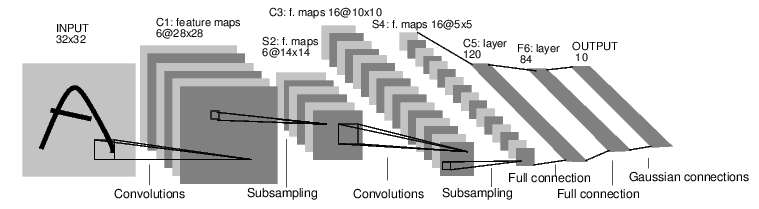1.定义网络

import torch
import torch.nn as nn
import torch.nn.functional as F

class Net(nn.Module):
def __init__(self):
super(Net, self).__init__()
#卷积层， '1'表示输入图片的为单通道，'6'表示输出通道数，'5'表示卷积核 5*5
#核心
self.conv1 = nn.Conv2d(1, 6, 5)
# '6'表示输入数据通道，16个输出，'5'表示卷积核 5*5
self.conv2 = nn.Conv2d(6, 16, 5)

#映射层/全连接层：y = Wx+b
# 为什么是16*5*5呢？ 这是全连接层的第一个函数。
# 经过卷积、池化后最后输出的结果是16个大小为5*5的图像.
#第一次卷积：图片大小 = （32-5+1）/1 = 28
#第一次池化：图片大小 = 28/2 = 14
#第二次卷积：图片大小 = （14-5+1）/1 =10
#第二次池化：图片大小 = 10/2 = 5
self.fc1 = nn.Linear(16 * 5 * 5,120)
self.fc2 = nn.Linear(120,84)
self.fc3 = nn.Linear(84,10)

def forward(self,x):
#在由多个输入平面组成哦输入信号上应用2D最大池化。
#(2,2)表示的是池化操作的步幅
x = F.max_pool2d(F.relu(self.conv1(x)),(2,2))
#如果大小是正方形，则只能指定一个数字
x = F.max_pool2d(F.relu(self.conv2(x)),2)
#将一个多维数据转成N行一列的数据。
x = x.view(-1,self.num_flat_features(x))
x = F.relu(self.fc1(x))
x = F.relu(self.fc2(x))
x =self.fc3(x)
return x

def num_flat_features(self,x):
size = x.size()[1:]
num_features = 1
for s in size:
num_features *= s
return num_features

net = Net()
print(net)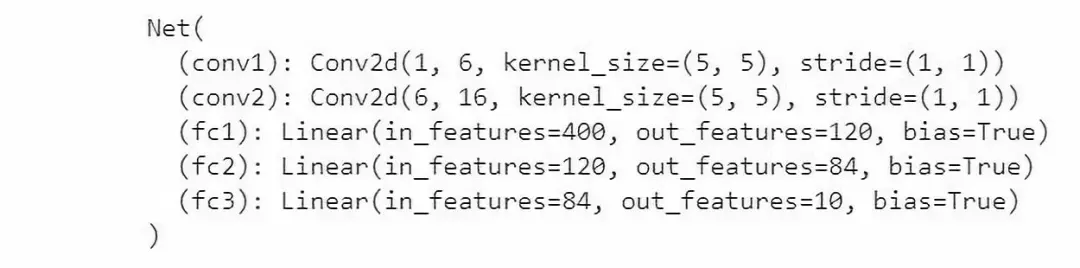params = list(net.parameters())
print(len(params))
print(params.size())   # conv1's.weight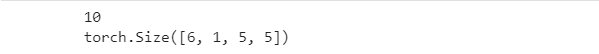input = torch.randn(1, 1, 32, 32)
out = net(input)
print(out)

out.backward(torch.randn(1,10))

torch.nn 只支持小批量数据。所有的torch.nn包都只支持输入样本为总样本的一小批，不支持单个样本。

torch.Tensor 多维数组支持自动求导操作与backward()类似。

nn.Module 神经网络模块。方便的方式封装参数, 帮助将其移动到GPU, 导出, 加载等.

nn.Parameter Tensor的一种，在被指定为 Module 的属性时，会自动被注册为一个参数。

autograd.Function 实现 autograd 操作的向前和向后定义..每个 Tensor 操作, 至少创建一个 Function 节点, 连接到创建 Tensor的函数, 并编码它的历史

2.损失函数

output = net(input)
target = torch.randn(10)  # a dummy target, for example
target = target.view(1, -1)  # make it the same shape as output
criterion = nn.MSELoss()

loss = criterion(output, target)
print(loss)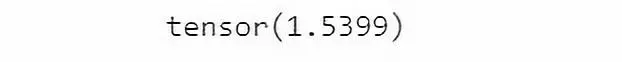input -> conv2d -> relu -> maxpool2d -> conv2d -> relu -> maxpool2d -> view -> linear -> relu -> linear -> relu -> linear -> MSELoss -> loss3.反向传播

net.zero_grad()     # zeroes the gradient buffers of all parameters

loss.backward()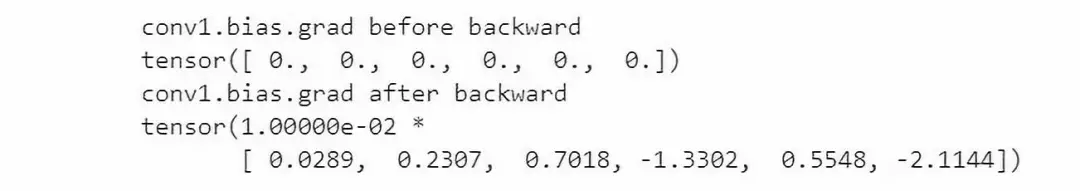4.更新权重

weight = weight - learning_rate * gradient

learning_rate = 0.01
for f in net.parameters():

import torch.optim as optim

#构建优化器
optimizer = optim.SGD(net.parameters(), lr=0.01)

#训练时循环的语句: